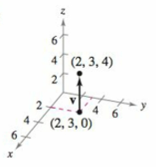Chapter 11.2, Problem 48E

Chapter
Section
Textbook Problem

# Finding the Component Form of a Vector in Space In Exercises 45-48, (a) find the component form of the vector v, (b) write the vector using standard unit vector notation, and (c) Sketch the vector with its initial point at the origin.(a)

To determine

To calculate: The component form of the vector v with the help of the graph provided in the question.

Explanation

Given:

Refer to the graph provided in the question.

Formula used:

The component form for any vector x directed by a line segment with end points P=(p1,p2,p3) and Q=(q1,q2,q3) is:

x=v1,v2,v3=q1p1,q2p2,q3p3

Calculation:

Refer to the graph provided in question to find out the endpoints, that are: (2,3,4),(2,3,0)

(b)

To determine

To calculate: The standard unit notation of the vector v=0,0,4.

(c)

To determine

To graph: The vector v=4k with initial point at (0,0,0).

### Still sussing out bartleby?

Check out a sample textbook solution.

See a sample solution

#### The Solution to Your Study Problems

Bartleby provides explanations to thousands of textbook problems written by our experts, many with advanced degrees!

Get Started

#### In Exercises 107-120, factor each expression completely. 118. 8a2 2ab 6b2

Applied Calculus for the Managerial, Life, and Social Sciences: A Brief Approach

#### Evaluate the integrals in Problems 5-28. Check your answers by differentiating. 16.

Mathematical Applications for the Management, Life, and Social Sciences

#### In Exercises 110, evaluate the expression. 20!

Finite Mathematics for the Managerial, Life, and Social Sciences

#### Find for .

Study Guide for Stewart's Multivariable Calculus, 8th

#### Simplify each expression. i100

College Algebra (MindTap Course List)